# Multiplication Multi-Step Word Problems

##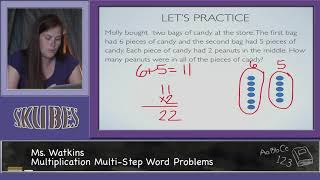By Skubes ed

Solve multistep word problems posed with whole numbers and having whole-number answers using the four operations# [4.OA.3-1.0] Multi-step Word Problems - Common Core Standard

##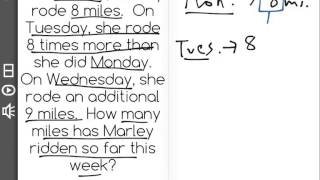By Freckle education

Solve multistep word problems posed with whole numbers and having whole-number answers using the four operations# Box Multiplication: Partial Products

##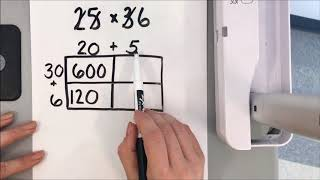By Erica Carmody

Multiply a whole number of up to four digits by a one-digit whole number, and multiply two two-digit numbers, using strategies based on place value and the properties of operations# Two-Digit by Two Digit Multiplication with Area Models: Grade 4

##By University Of Houstan Mathematics Education

Multiply a whole number of up to four digits by a one-digit whole number, and multiply two two-digit numbers, using strategies based on place value and the properties of operations.# Two-Digit by Two-Digit Multiplication: Grade 4

##By University Of Houstan Mathematics Education

Multiply a whole number of up to four digits by a one-digit whole number, and multiply two two-digit numbers, using strategies based on place value and the properties of operations.# MAFS.3.NBT.1.3

##By Brittany Bezada

We are multiplying one-digit whole numbers by multiples of 10 in the range 10-90 using strategies based on place value and properties of operations.# Multiplying by 10

##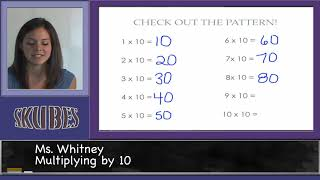By Skubes ed

Multiply one-digit whole numbers by multiples of 10 in the range 10–90 (e.g., 9 × 80, 5 × 60) using strategies based on place value and properties of operations.# Ms. Chang 4th Grade- Divide with partial quotients

##By Ms Chang

Find whole-number quotients and remainders with up to four-digit dividends and one-digit divisors, using strategies based on place value, the properties of operations, and/or the relationship between multiplication and division# [4.NBT.6-1.0] Four digit division - Common Core Standard

##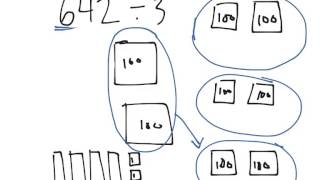By Freckle education

Find whole-number quotients and remainders with up to four-digit dividends and one-digit divisors, using strategies based on place value, the properties of operations, and/or the relationship between multiplication and division# Third Grade Math|‚ New York State Common Core|‚ Operations and Algebraic Thinking|”‚ No (1) Counting

##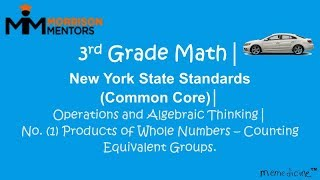By Jacy Campbell

- 3.OA.A.1 Interpret products of whole numbers (i.e. interpret 5 × 7 as the total number of objects in 5 groups of 7 objects each)# Grade 3 Math - Understanding Division

##By Lumos Learning

Using the Lumos Study Programs, parents and educators can reinforce the classroom learning experience for children and help them succeed at school and on the standardized tests. Lumos books, dvd, eLearning and tutoring are used by leading schools, libraries and thousands of parents to supplement classroom learning and improve student achievement in the standardized tests.

Tags: Operations & Algebraic Thinking, whole numbers, 3.OA.A.2, standardized tests# [4.NBT.5-1.0] Four digit multiplication - Common Core Standard - Word Problem

##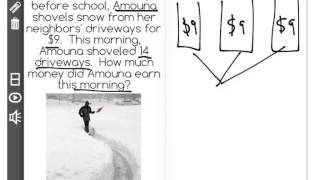By Freckle Education

Discover more Common Core Math at https://www.frontrowed.comMultiply a whole number of up to four digits by a one-digit whole number using strategies based on place value and the properties of operations. Illustrate and explain the calculation by using equations, rectangular arrays, and/or area models.Front Row is a free, adaptive, Common Core aligned math program for teachers and students in kindergarten through eighth grade. Front Row allows students to practice math at their own pace - learning advanced concepts when they 're ready and receiving remediation when they struggle. Front Row provides teachers with access to a detailed data dashboard and weekly email reports that show which standards are causing students difficulty, what small groups can be formed for interventions, and how their students are progressing in math.Discover more Common Core Math at https://www.frontrowed.com# [5.NBT.6-1.0] Multi-digit divisors - Common Core Standard - Word Problem

##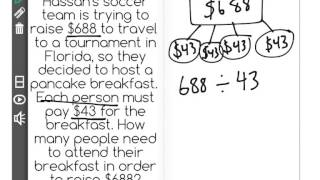By Front Row

Discover more Common Core Math at https://www.frontrowed.com Find whole-number quotients of whole numbers with up to four-digit dividends and two-digit divisors, using strategies based on place value, the properties of operations, and/or the relationship between multiplication and division Front Row is a free, adaptive, Common Core aligned math program for teachers and students in kindergarten through eighth grade. Front Row allows students to practice math at their own pace - learning advanced concepts when they're ready and receiving remediation when they struggle. Front Row provides teachers with access to a detailed data dashboard and weekly email reports that show which standards are causing students difficulty, what small groups can be formed for interventions, and how their students are progressing in math.Discover more Common Core Math at https://www.frontrowed.com# [7.EE.3-1.0] Solve Multi-Step Problems - Common Core Standard

##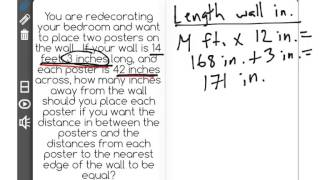By Front Row

Discover more Common Core Math at https://www.frontrowed.comSolve multi-step mathematical problems posed with positive and negative rational numbers in any form (whole numbers, fractions, and decimals), using tools strategically. Apply properties of operations to calculate with numbers in any form; convert between forms as appropriate.Front Row is a free, adaptive, Common Core aligned math program for teachers and students in kindergarten through eighth grade. Front Row allows students to practice math at their own pace - learning advanced concepts when they're ready and receiving remediation when they struggle. Front Row provides teachers with access to a detailed data dashboard and weekly email reports that show which standards are causing students difficulty, what small groups can be formed for interventions, and how their students are progressing in math.Discover more Common Core Math at https://www.frontrowed.com# Even and Odd Numbers - Basic Introduction

##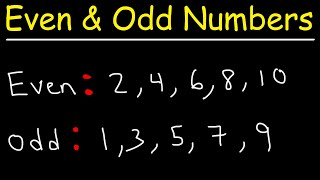By The Organic Chemistry Tutor# [3.NBT.3-1.0] Intro multiplication - Common Core Standard

##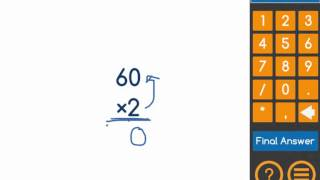By Freckle by Renaissance

Multiply one-digit whole numbers by multiples of 10 in the range 10–90 (e.g., 9 × 80, 5 × 60) using strategies based on place value and properties of operations. Front Row is a free, adaptive, Common Core aligned math program for teachers and students in kindergarten through eighth grade. Front Row allows students to practice math at their own pace - learning advanced concepts when they're ready and receiving remediation when they struggle. Front Row provides teachers with access to a detailed data dashboard and weekly email reports that show which standards are causing students difficulty, what small groups can be formed for interventions, and how their students are progressing in math.# Math Antics - Adding and Subtracting Fractions

##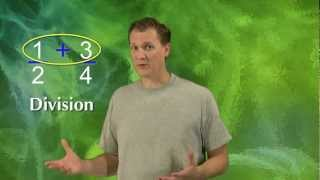By mathantics# Math Antics - Subtracting Mixed Numbers

##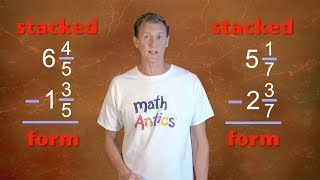By mathantics# Grade 5 Math Lessons and Worksheets

##By Lumos Learning

It is important for you as a parent to know what your child should be learning in grade 5 math. This is for you to be able to monitor your child’s progress and see if they are having any difficulty with the subject. If you supervise their learning process, then you can determine whether your child needs more help or tutoring. Having a clear idea of the math concepts that your child should be learning also gives you a chance to reinforce their knowledge. Familiarize yourself with the lessons that he or she is learning about so that you can help them anytime they need your assistance. The major topics that are included in grade 5 math are math strands involving whole numbers, decimals, fractions, measurement, plane figures, area and perimeter, ratio, scale, percent, average, graphs, rectangular prisms and cubes, volume, rates and speed, and functions and equations. Your child should develop competency in understanding problems concerning these math concepts. Your child should also be able to translate that knowledge into their problem solving skills. These problems should be applicable in the real world so that your child will be able to visualize and analyze the situation. Grade 5 math already includes using formulas and creating solutions for math problems. This is the introduction of the more complex math concepts and operations. It is preferable that you guide and aid your child as much as you can at this stage. The danger of having your child experience too much difficulty in the subject is that he or she will completely lose interest in math. You should try to avoid this and help your child develop a strong foundation in math concepts. As your child goes on to learn about harder math, you should also be more willing to give them assistance.# Can you solve this tricky math crossword?

##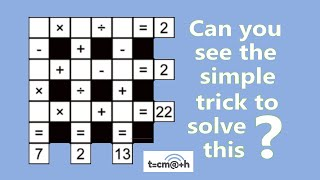By tecmath